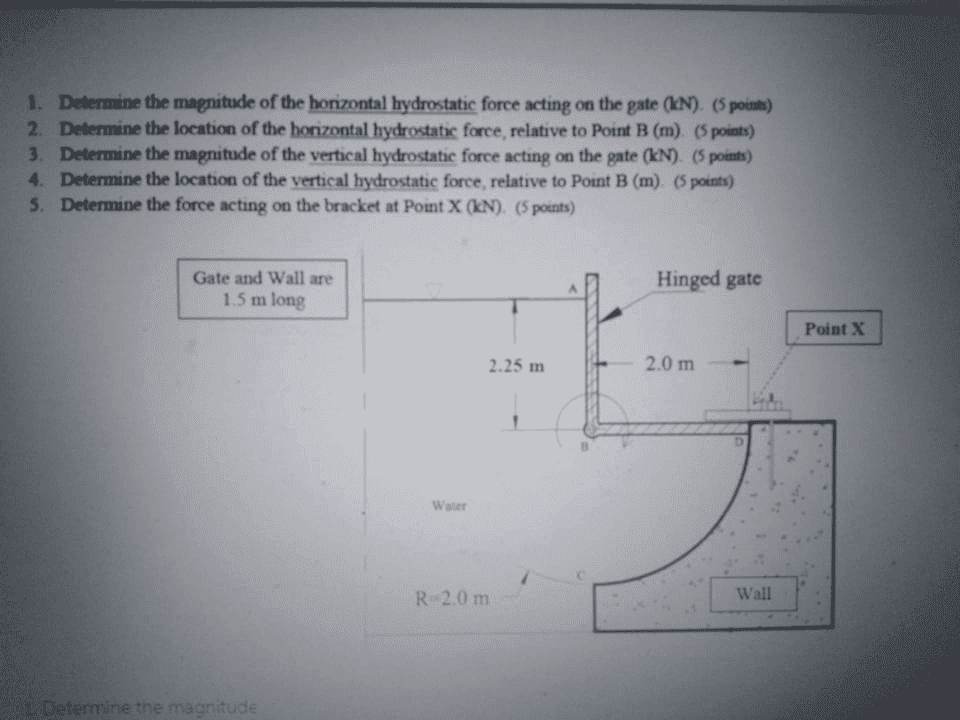# Fluid mechanics problem: Hydrostatic forces acting on a gate

civil_eng_student
Homework Statement:
hydrostatic forces acting on gate,need to find the force at point X in order to have equilibrium
Relevant Equations:
I posted this because i used two methods in order to find the force acting at point X but i get different results...
my thoughts:
in order to find the force at X i just need to ΣΜ(B)=0 so i just need to find the forces acting on ABD.
first on AB i found the hydrostatic force =(1/2)*2,25*γ*2,25*length and the distance from b is 0,75m.
secondly we have a uniformly load acting on BD=2,25*γ*2*length and distance 1 from b.
under ABD we have a quarter of a circle so i know that the forces acts on B(the center) so they dont give any moments...
then i sum the moments and find the force.
now using the second method is finding the forces acting on the quarter and not putting them acting on the center.
for the horizontal force i find F1=2,25*γ*R*lenght and F2=(1/2)*2*γ*R*length. F1 is acting 1 from b and F2 4/3.
then the vertical acts on the centroid and the distance is 4*R/3*PI=0,848.
then i sum the moments and find a different result for the force a X.
hope i was clear.Last edited by a moderator: